# Similarities and Differences among RANK, DENSE_RANK and ROW_NUMBER Functions

Total: 65 Average: 3.8

The RANK, DENSE_RANK, and ROW_NUMBER functions are used to retrieve an increasing integer value. They start with a value based on the condition imposed by the ORDER BY clause. All of these functions require the ORDER BY clause to function properly. In the case of partitioned data, the integer counter is reset to 1 for each partition.

In this article, we will study the RANK, DENSE_RANK, and ROW_NUMBER functions in detail, but before that, let’s create dummy data that these functions can be used on unless your database is fully backed up.

## Preparing Dummy Data

Execute the following script to create a database called ShowRoom and containing a table called Cars (that contains 15 random records of cars):

```CREATE Database ShowRoom;
GO
USE ShowRoom;

CREATE TABLE Cars
(
id INT,
name VARCHAR(50) NOT NULL,
company VARCHAR(50) NOT NULL,
power INT NOT NULL
)

USE ShowRoom
INSERT INTO Cars
VALUES
(1, 'Corrolla', 'Toyota', 1800),
(2, 'City', 'Honda', 1500),
(3, 'C200', 'Mercedez', 2000),
(4, 'Vitz', 'Toyota', 1300),
(5, 'Baleno', 'Suzuki', 1500),
(6, 'C500', 'Mercedez', 5000),
(7, '800', 'BMW', 8000),
(8, 'Mustang', 'Ford', 5000),
(9, '208', 'Peugeot', 5400),
(10, 'Prius', 'Toyota', 3200),
(11, 'Atlas', 'Volkswagen', 5000),
(12, '110', 'Bugatti', 8000),
(13, 'Landcruiser', 'Toyota', 3000),
(14, 'Civic', 'Honda', 1800),
(15, 'Accord', 'Honda', 2000)```

## RANK Function

The RANK function is used to retrieve ranked rows based on the condition of the ORDER BY clause. For example, if you want to find the name of the car with the third highest power, you can use the RANK function.
Let’s see RANK Function in action:

```SELECT name,company, power,
RANK() OVER(ORDER BY power DESC) AS PowerRank
FROM Cars```

The script above finds and ranks all the records in the Cars table and orders them in order of descending power. The output looks like this: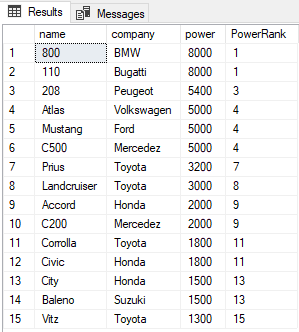The PowerRank column in the above table contains the RANK of the cars ordered by descending order of their power. An interesting thing about the RANK function is that if there is a tie between N previous records for the value in the ORDER BY column, the RANK functions skips the next N-1 positions before incrementing the counter. For instance, in the above result, there is a tie for the values in the power column between the 1st and 2nd rows, therefore the RANK function skips the next (2-1 = 1) one record and jumps directly to the 3rd row.
The RANK function can be used in combination with the PARTITION BY clause. In that case, the rank will be reset for each new partition. Take a look at the following script:

```SELECT name,company, power,
RANK() OVER(PARTITION BY company ORDER BY power DESC) AS PowerRank
FROM Cars```

In the script above, we partition the results by the company column. Now for each company, the RANK will be reset to 1 as shown below: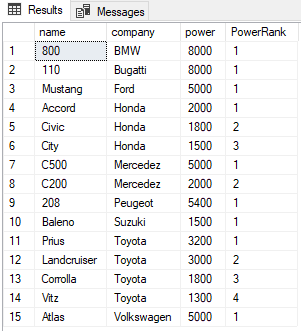## DENSE_RANK Function

The DENSE_RANK function is similar to the RANK function however the DENSE_RANK function does not skip any ranks if there is a tie between the ranks of the preceding records. Take a look at the following script.

```SELECT name,company, power,
RANK() OVER(PARTITION BY company ORDER BY power DESC) AS PowerRank
FROM Cars```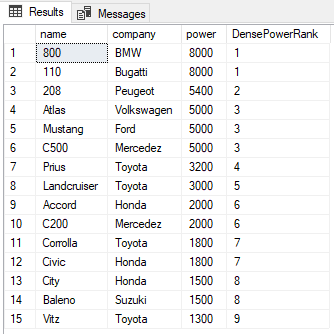You can see from the output that despite there being a tie between the ranks of the first two rows, the next rank is not skipped and has been assigned a value of 2 instead of 3. As with the RANK function, the PARTITION BY clause can also be used with the DENSE_RANK function as shown below:

```SELECT name,company, power,
DENSE_RANK() OVER(PARTITION BY company ORDER BY power DESC) AS DensePowerRank
FROM Cars```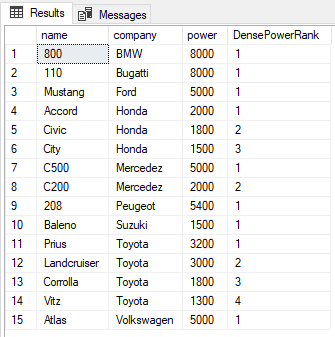## ROW_NUMBER Function

Unlike the RANK and DENSE_RANK functions, the ROW_NUMBER function simply returns the row number of the sorted records starting with 1. For example, if RANK and DENSE_RANK functions of the first two records in the ORDER BY column are equal, both of them are assigned 1 as their RANK and DENSE_RANK. However, the ROW_NUMBER function will assign values 1 and 2 to those rows without taking the fact that they are equally into account. Execute the following script to see the ROW_NUMBER function in action.

```SELECT name,company, power,
ROW_NUMBER() OVER(ORDER BY power DESC) AS RowRank
FROM Cars```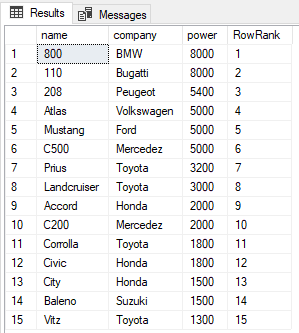From the output, you can see that the ROW_NUMBER function simply assigns a new row number to each record irrespective of its value.
The PARTITION BY clause can also be used with the ROW_NUMBER function as shown below:

```SELECT name, company, power,
ROW_NUMBER() OVER(PARTITION BY company ORDER BY power DESC) AS RowRank
FROM Cars```

The output looks like this: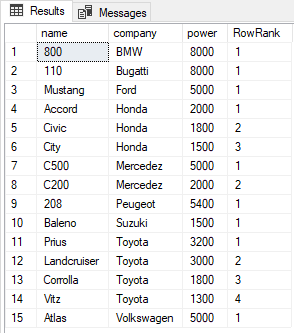## Similarities between RANK, DENSE_RANK, and ROW_NUMBER Functions

The RANK, DENSE_RANK, and ROW_NUMBER functions have the following similarities:
1 – All of them require an order by clause.
2 – All of them return an increasing integer with a base value of 1.
3 – When combined with a PARTITION BY clause, all of these functions reset the returned integer value to 1 as we have seen.
4 – If there are no duplicated values in the column used by the ORDER BY clause, these functions return the same output.
To illustrate the last point, let’s create a new table Car1 in the ShowRoom database with no duplicate values in the power column. Execute the following script:

```USE ShowRoom;

CREATE TABLE Cars1
(
id INT,
name VARCHAR(50) NOT NULL,
company VARCHAR(50) NOT NULL,
power INT NOT NULL
)

INSERT INTO Cars1
VALUES
(1, 'Corrolla', 'Toyota', 1800),
(2, 'City', 'Honda', 1500),
(3, 'C200', 'Mercedez', 2000),
(4, 'Vitz', 'Toyota', 1300),
(5, 'Baleno', 'Suzuki', 2500),
(6, 'C500', 'Mercedez', 5000),
(7, '800', 'BMW', 8000),
(8, 'Mustang', 'Ford', 4000),
(9, '208', 'Peugeot', 5400),
(10, 'Prius', 'Toyota', 3200)
The cars1 table has no duplicate values. Now let’s execute the RANK, DENSE_RANK and ROW_NUMBER functions on the Cars1 table ORDER BY power column. Execute the following script:
SELECT name,company, power,
RANK() OVER(ORDER BY power DESC) AS [Rank],
DENSE_RANK() OVER(ORDER BY power DESC) AS [Dense Rank],
ROW_NUMBER() OVER(ORDER BY power DESC) AS [Row Number]
FROM Cars1```

The output looks like this: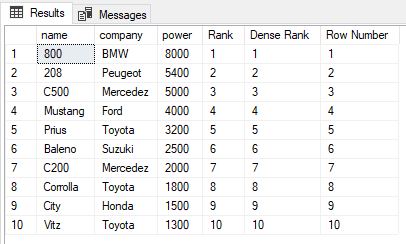You can see that there are no duplicate values in the power column which is being used in the ORDER BY clause, therefore the output of the RANK, DENSE_RANK, and ROW_NUMBER functions are the same.

## Difference between RANK, DENSE_RANK, and ROW_NUMBER Functions

The only difference between RANK, DENSE_RANK, and ROW_NUMBER function is when there are duplicate values in the column being used in the ORDER BY Clause.
If you go back to the Cars table in the ShowRoom database, you can see it contains lots of duplicate values. Let’s try to find the RANK, DENSE_RANK, and ROW_NUMBER of the Cars1 table ordered by power. Execute the following script:
SELECT name,company, power,

```RANK() OVER(ORDER BY power DESC) AS [Rank],
DENSE_RANK() OVER(ORDER BY power DESC) AS [Dense Rank],
ROW_NUMBER() OVER(ORDER BY power DESC) AS [Row Number]
FROM Cars```

The output looks like this: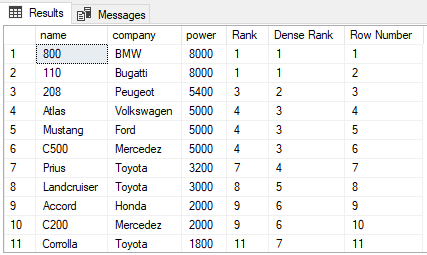From the output, you can see that the RANK function skips the next N-1 ranks if there is a tie between N previous ranks. On the other hand, the DENSE_RANK function does not skip ranks if there is a tie between ranks. Finally, the ROW_NUMBER function has no concern with ranking. It simply returns the row number of the sorted records. Even if there are duplicate records in the column used in the ORDER BY clause, the ROW_NUMBER function will not return duplicate values. Instead, it will continue to increment irrespective of the duplicate values.